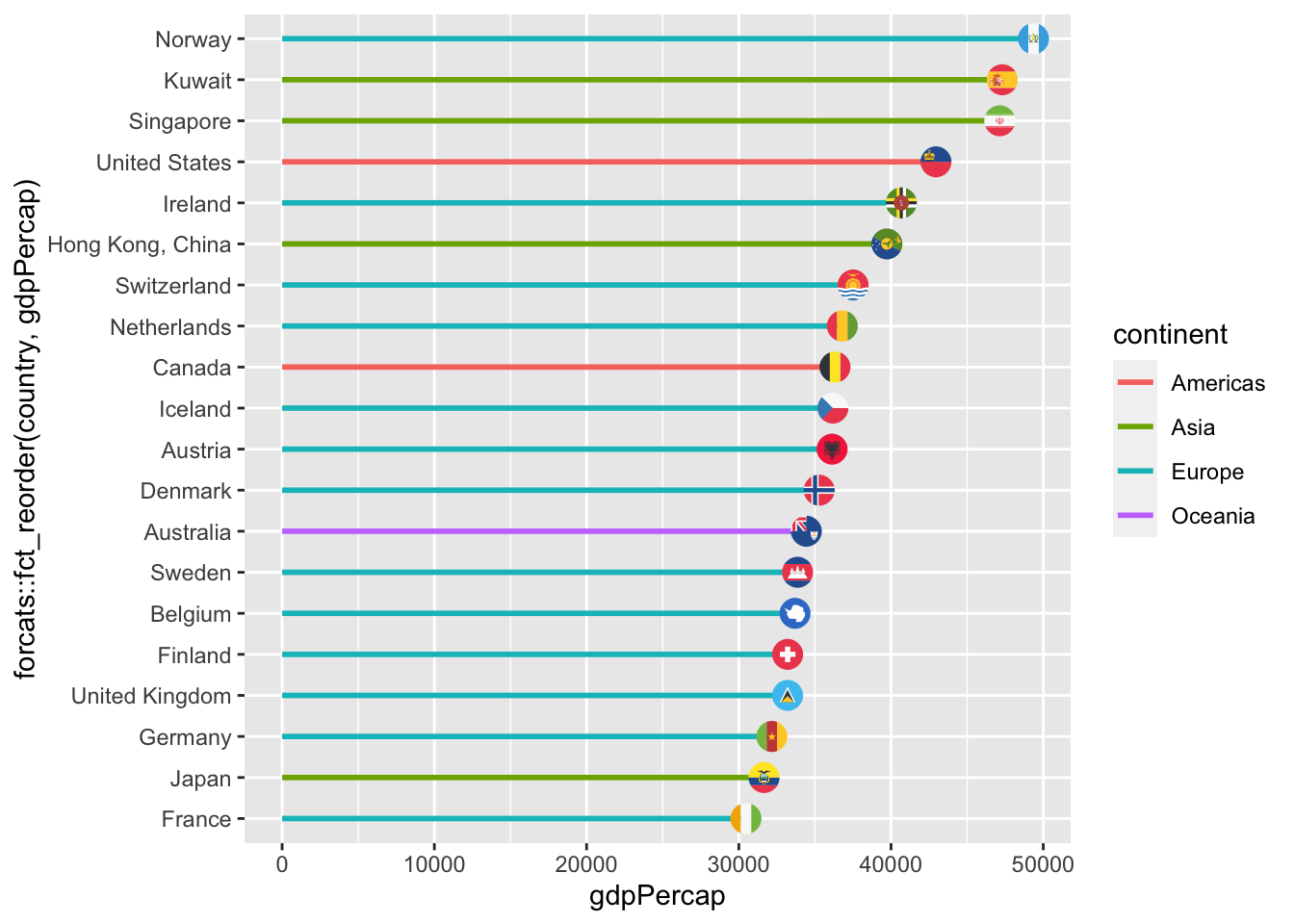# 1.3 — Data Visualization with ggplot2 — Class Notes

## Contents

Tuesday, August 25, 2020

## Overview

Today we start the fun stuff - data visualization. We will cover how to build plots with the package `ggplot2` (part of the `tidyverse`): I will lecture for the first half (again, it will be a lot of information that you can refer back to as needed over the semester) and you will practice making plots.

## Live Class Session on Zoom

The live class Zoom meeting link can be found on Blackboard (see `LIVE ZOOM MEETINGS` on the left navigation menu), starting at 11:30 AM.

If you are unable to join today’s live session, or if you want to review, you can find the recording stored on Blackboard via Panopto (see `Class Recordings` on the left navigation menu).

## Practice Problems

Answers from last class’ practice problems on base R are posted on that page. Today you will be working on R practice problems on data visualization. Answers will be posted later.

## Statistics and Software Survey

“Answers” for the statistics survey have been posted, including some summaries of how your classmates feel.

## Assignments

None!

### New Packages Mentioned

Check back here later for more tips on plotting with ggplot2!

### Extensions to ggplot2

`ggplot2`, being one of the most popular packages, has a lot of user-made extensions that allow you to do lots of neat things with your plots, from plotting networks, Parliaments, dendrograms, and other types of graphs, to formatting and visual tools that help improve your figures.

For the following demonstrations, we will use the `gapminder` data. Let’s start just by making a basic graph and saving it as an object called `p`. I have decided to map (`aes()`) each `geom_point`’s `color` to `continent` and `size` to `pop`:

``````library(gapminder)
library(ggplot2)

p<-ggplot(gapminder) +
aes(x = gdpPercap,
y = lifeExp) +
geom_point(aes(size = pop,
color = continent))
p``````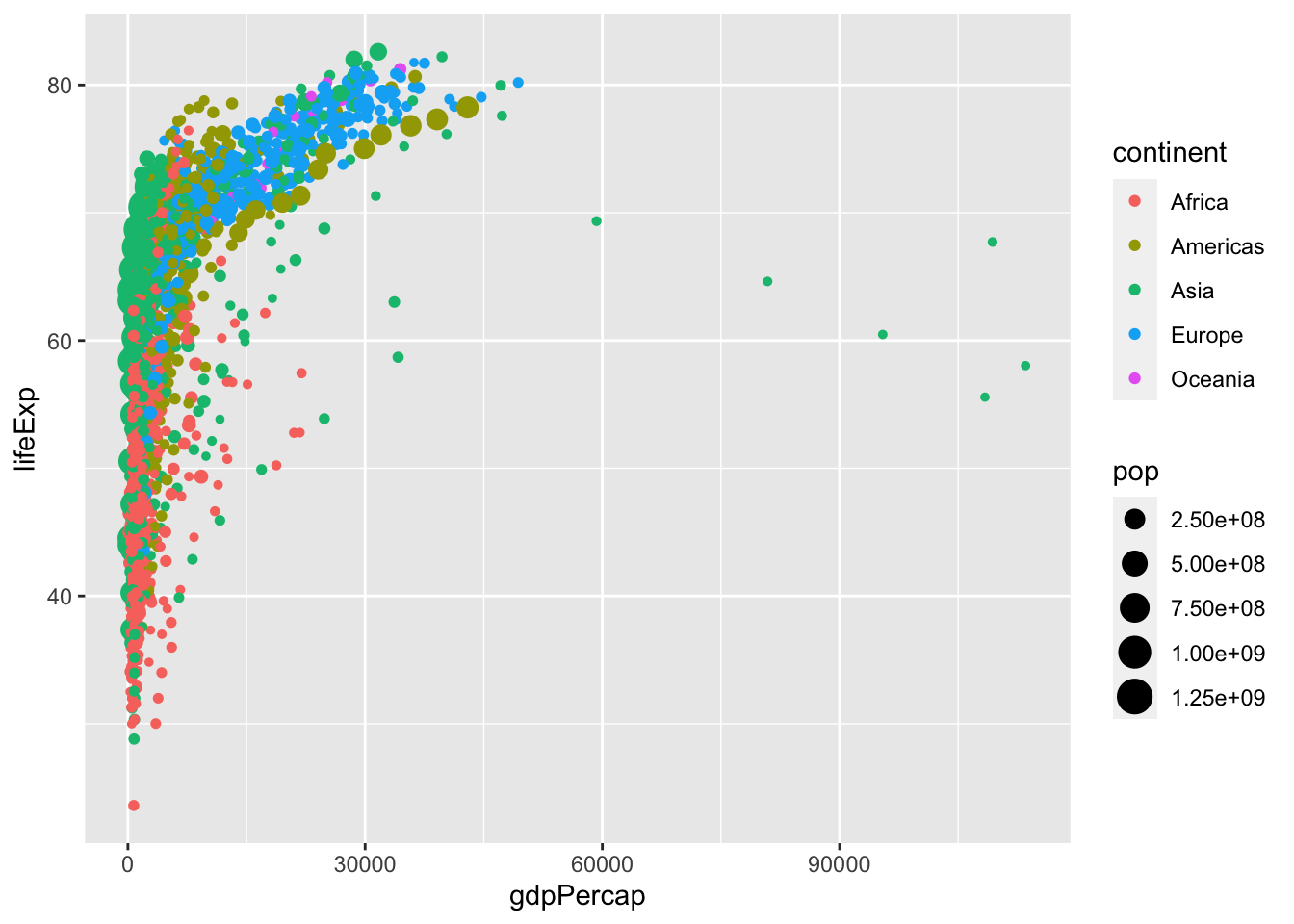#### Working with Scales

I don’t like the default choices `ggplot2` has made for my point sizes for population, or the way it depicts them (in scientific notation) on the legend.

I will set my own scale by setting the `breaks`The cut offs for using one size circle vs. the next/previous size circle, depending on population.

manually, according to a vector I define as: `c(100000, 1000000, 100000000, 1000000000)`. So, I will use one point size for populations of 100 thousand, a bigger one for a million, a bigger one for 100 millions, and the biggest for 1 billion.

I am going to `label` these (on my legend) as the following vector: `c("<1 million","1 million","100 million", "1 billion")`.

Lastly, I don’t think the size of the billion circle is big enough, a billion is a lot of people! So I will set the `range` of sizes from size `1` point to size `10` point.

To do this, I include all of this inside the `scale_size` command (because I am scaling the `size` of points):

``````# let's save this as p2
p2<-p+scale_size(breaks = c(100000, 1000000, 100000000, 1000000000), # cut offs
labels=c("<1 million","1 million","100 million", "1 billion"), # labels on legend
range=c(1,10)) # min & max point size

# let's see what we did
p2``````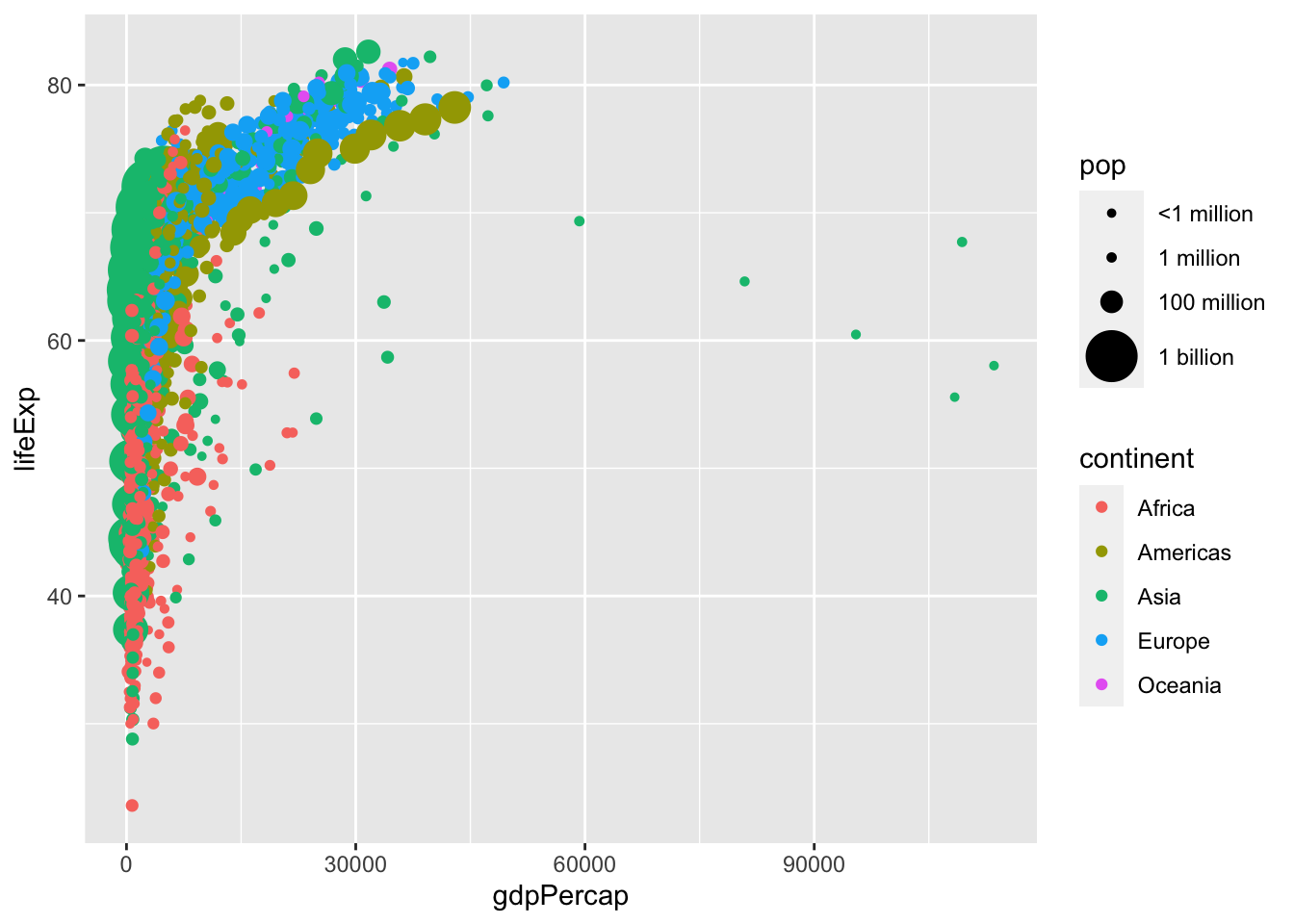This is also very hard to see the relationship (because it is nonlinear!). So I will rescale the `x_axis` logarithmically with base 10:

``p2+scale_x_log10()``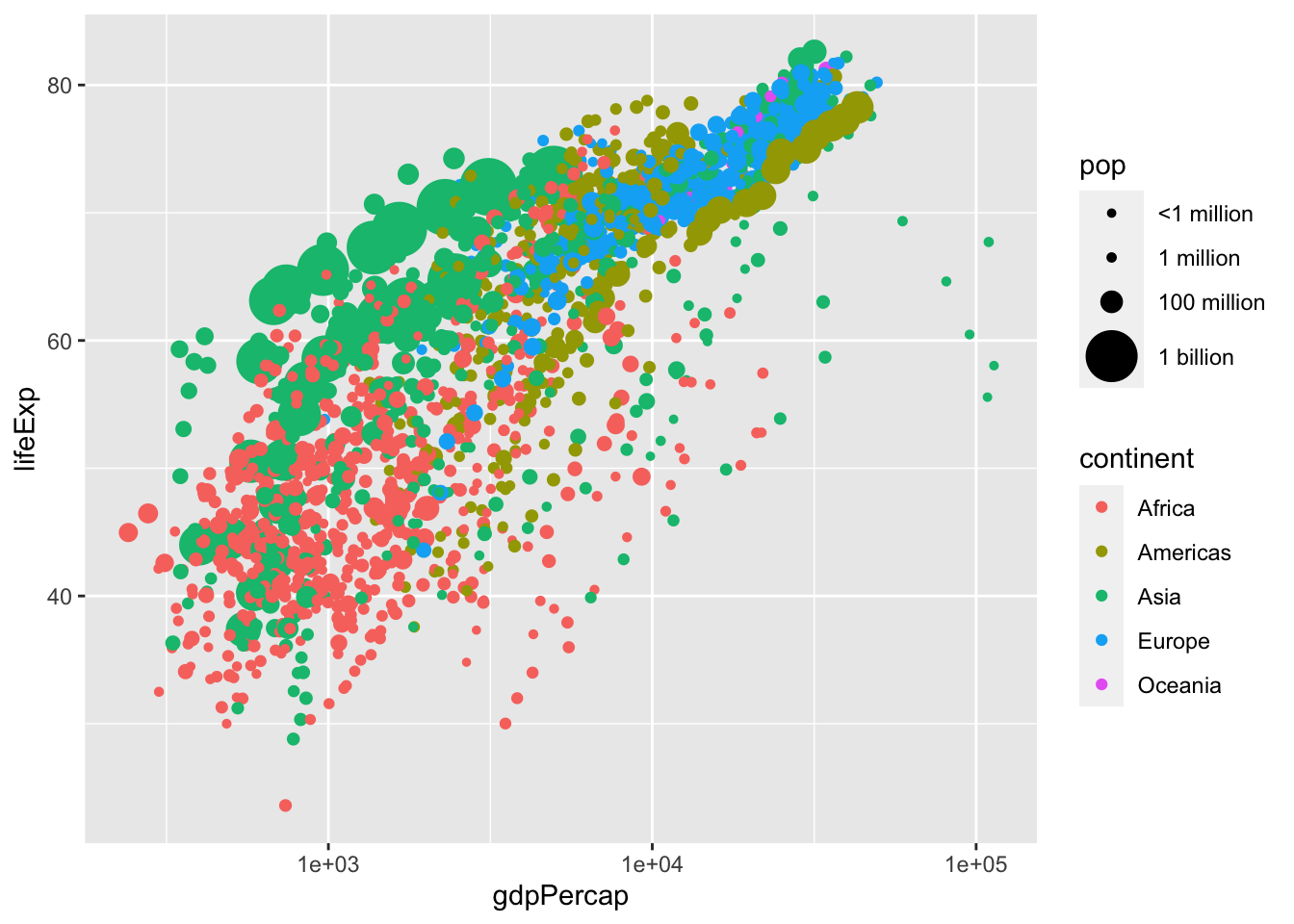Doing this already gives me a much clearer view of the relationship! But I don’t like the labels, or the breaks it has chosen, so I can customize them again:

``````p2+scale_x_log10(
breaks = c(10^3, 10^4, 10^5), # 1,000, 10,000, and 100,000
labels = scales::dollar)``````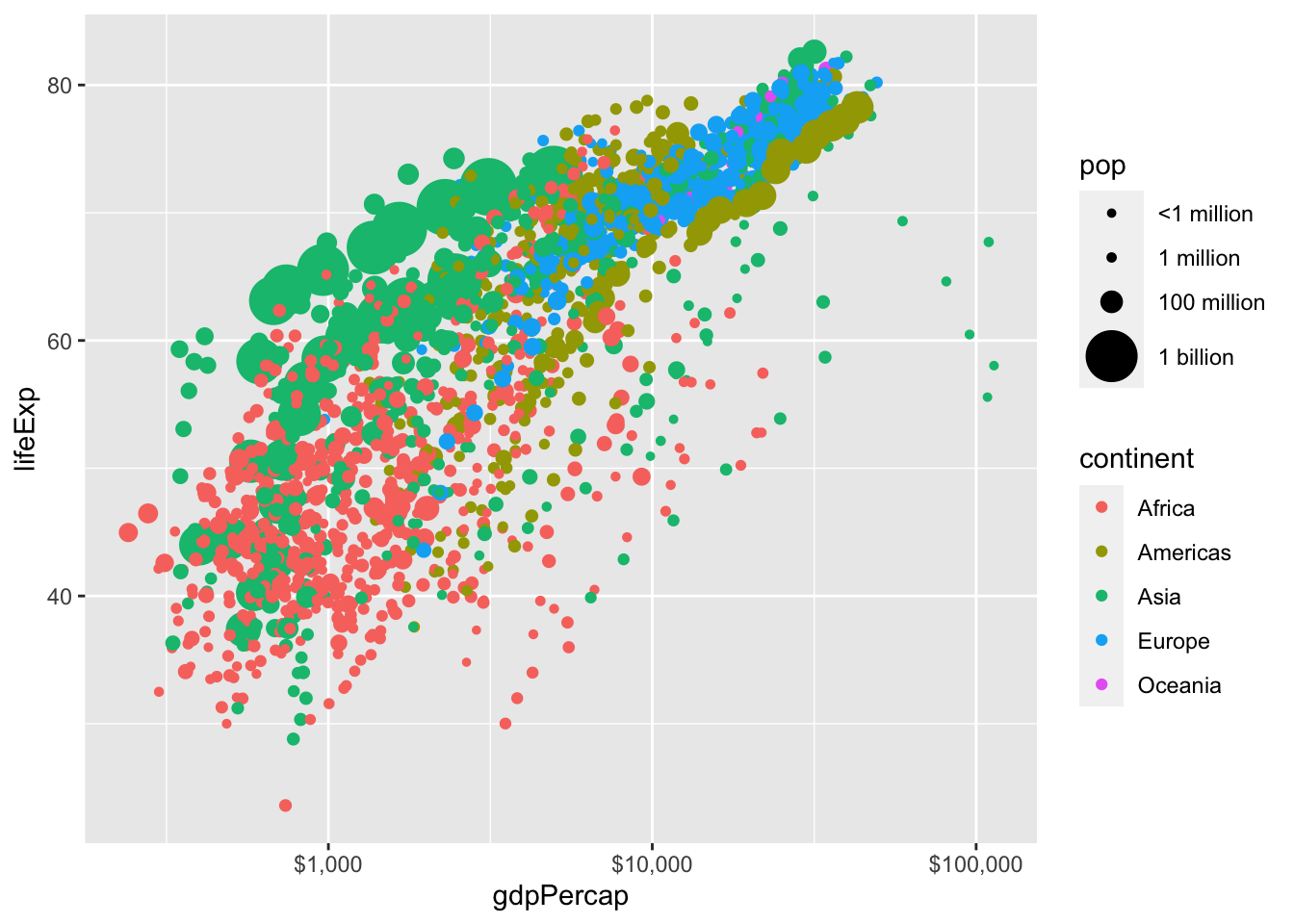The `scales` package has a nice command to format labels, in this case I am calling the `scales::dollar` function to print dollar signs in front of my axes numbers. I could have done it manually instead by setting `labels = c("\$1,000", "\$10,000", "\$100,000")`.

### Subsetting Data

We learn more about this in class 1.4 using `tidyverse`, but let’s only look at one year of data (there’s too much going on in this plot, especially with the large points, some points are covering other points). So let’s only look at the year 2007. I can do this in two ways:

1. Subset the data, save the subsetted data as an object (I’ll call `gap2007`), plot with that object as my data:
``library(tidyverse)``
``````gap2007 <- gapminder %>%
filter(year == 2007)

p2007 <- ggplot(data = gap2007)+
aes(x = gdpPercap,
y = lifeExp) +
geom_point(aes(size = pop,
color = continent))

p2007``````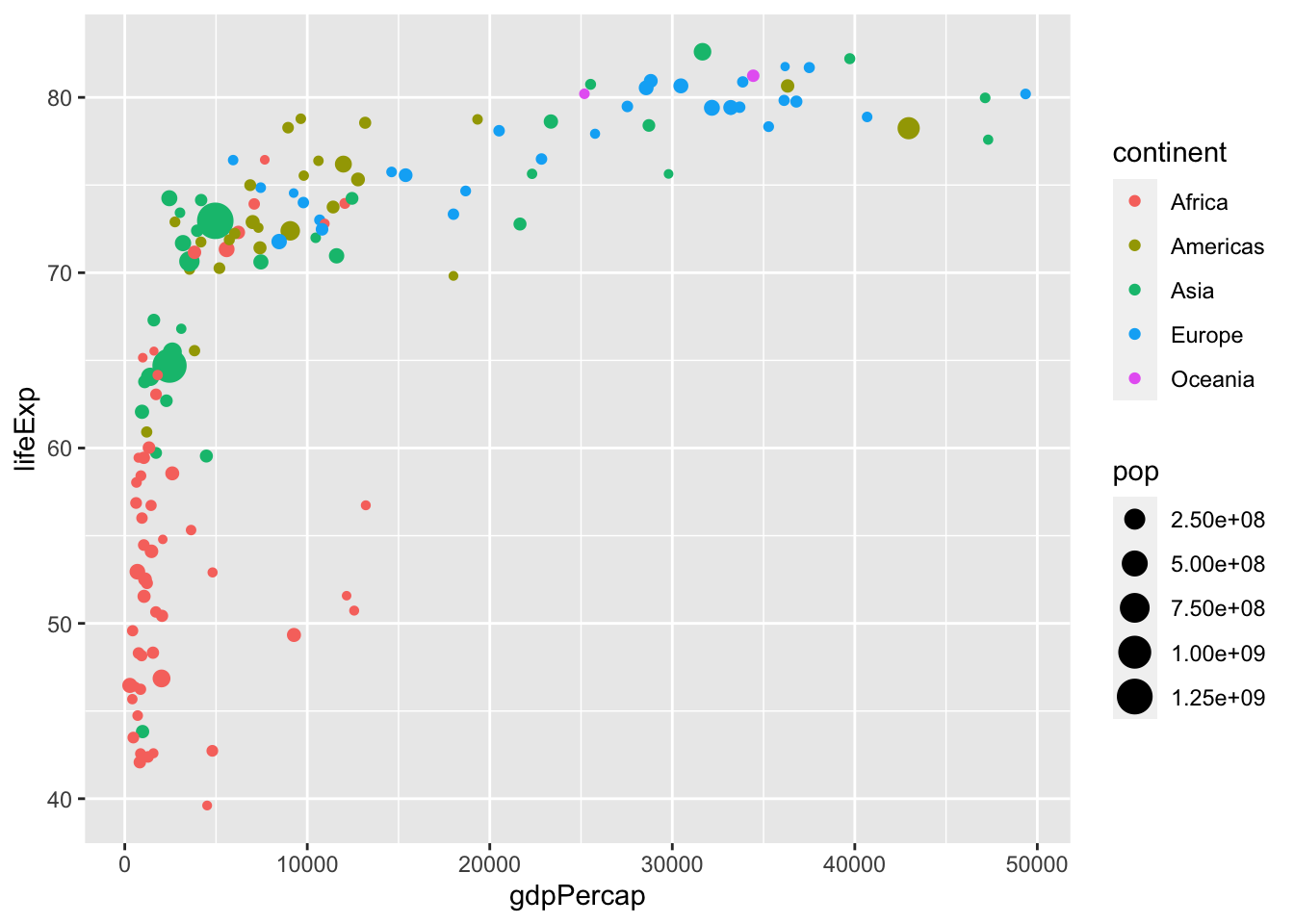1. Subset data and pipe it directly into `ggplot2`’s `data` argument:
``````library(tidyverse)

p2007 <- gapminder %>%
filter(year == 2007) %>%
ggplot(data = .)+ # . is placeholder
aes(x = gdpPercap,
y = lifeExp) +
geom_point(aes(size = pop,
color = continent))

p2007``````Now let’s clean up the graph with the same things I did before, hide the legends (set the `color` and `size`, my two `aes`thetic mappings, `guides` equal to `FALSE`), add some labels, and change the theme:

``````p3<-p2007+scale_size(breaks = c(100000, 1000000, 100000000, 1000000000),
labels=c("<1 million","1 million","100 million", "1 billion"),
range=c(1,10))+
scale_x_log10(
breaks = c(10^3, 10^4, 10^5),
labels = scales::dollar)+
labs(x = "GDP per Capita (USD)",
y = "Life Expectancy (years)")+
guides(color = FALSE,
size = FALSE)+
theme_classic()
p3``````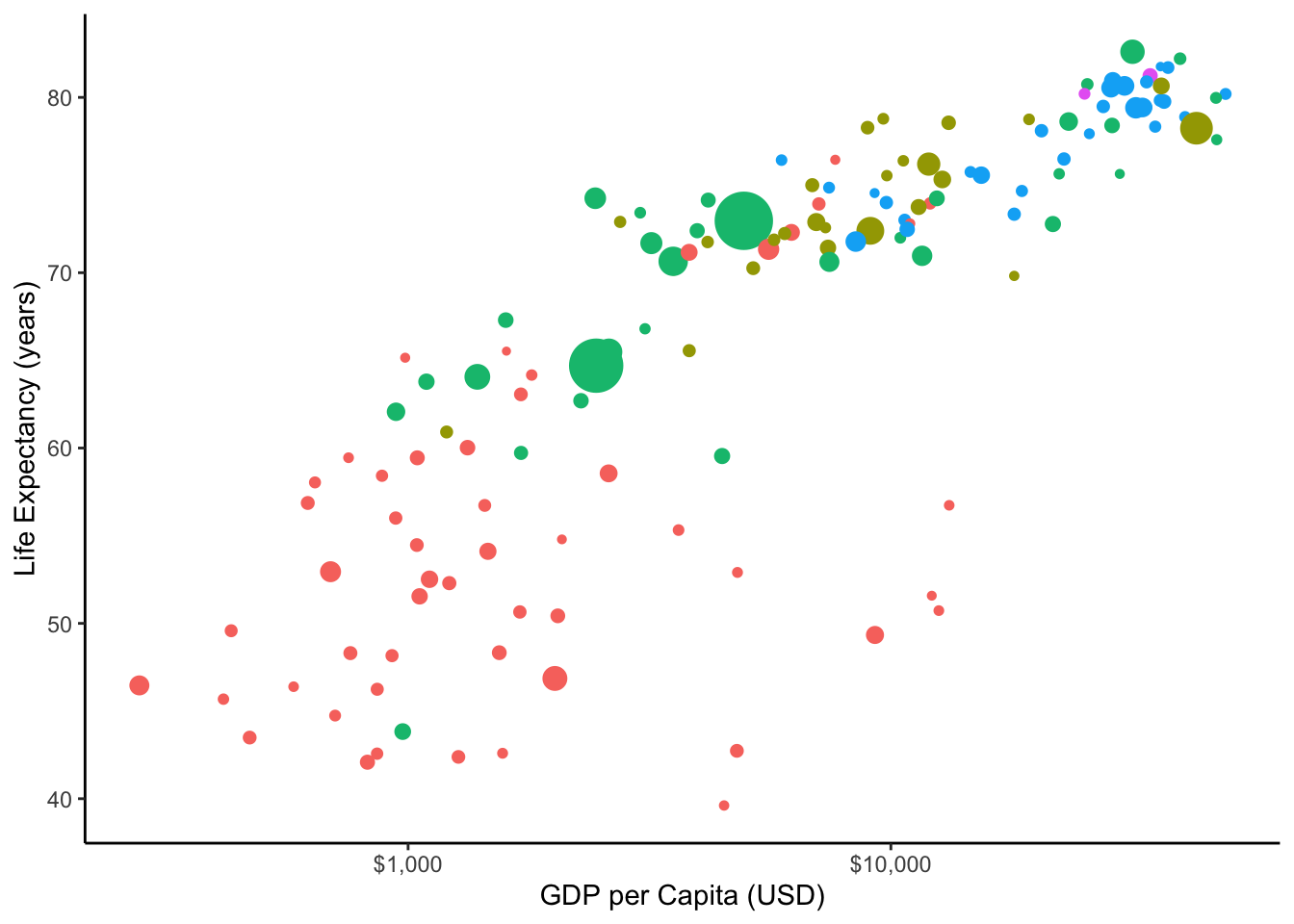### ggrepel

If I were to try to label some countries, with either `geom_text` (just word) or `geom_label()` (text in a box), setting the `label` `aes`thetic to `country`, see what would happen:

``````p3+geom_label(aes(label = country,
color = continent))``````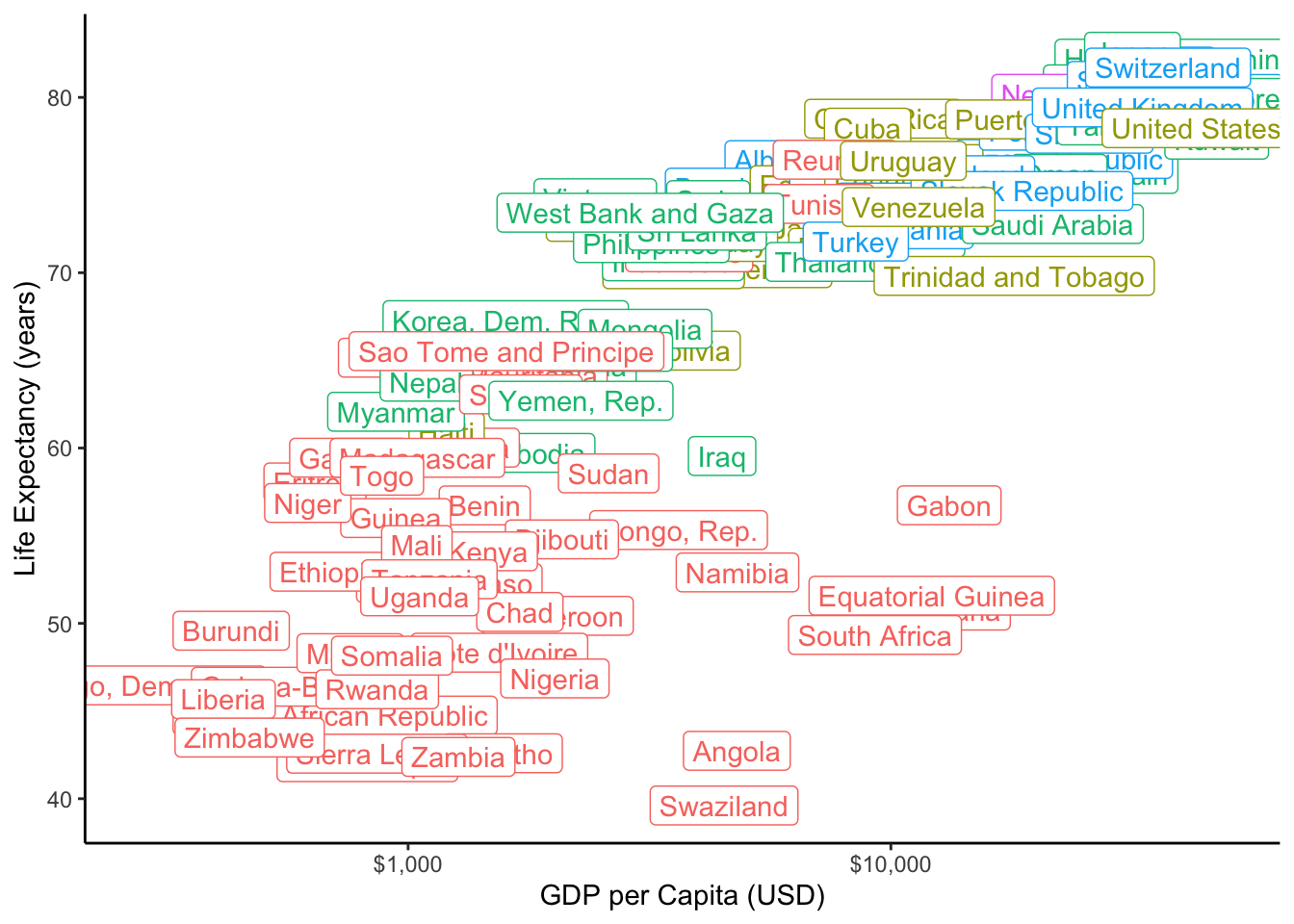The labels, which are plotted right on top of each point, cover the points!

Someone figured out a clever way to let us do both, and it is a package called `ggrepel`, which allows you to plot labels that are “repelled” away from the point they are labelling in an intelligent way. This is a separate package, which you must first install and then load with library to use it!

``````# install.packages("ggrepel") # do this only once
library(ggrepel)

p3+geom_text_repel(aes(label = country,
color = continent,
size = pop),
size = 3)``````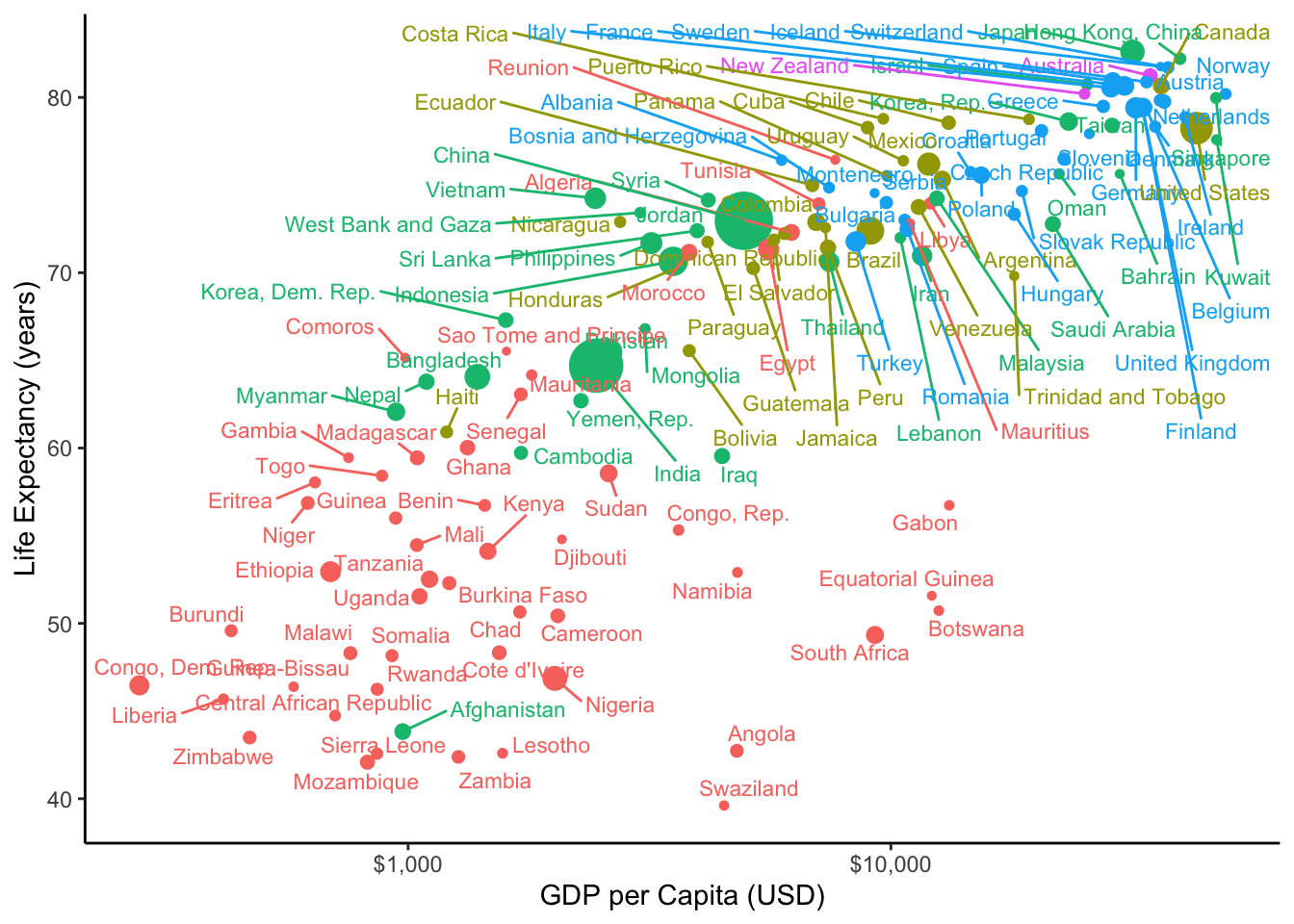This is much better, but for this particular chart, since a lot of observations are close together, it would be unwise to label everything, perhaps only label a subset of important points.

### ggflag

One alternative is instead of points, to use some other marking. Someone created the `ggflags` package to let you plot flags of countries. This creates a new type of geom, called `geom_flag`, that requires you to map the `country` `aes`thetic to a variable in your data with the country name (incidentally, in `gapminder` that variable is also called `country`). Let’s try that out instead (and add my same customizations as above):

``````# install.packages("ggflags") # do this only once
library(ggflags)
pflag<-p3+geom_flag(aes(country = country,
size = pop))
pflag``````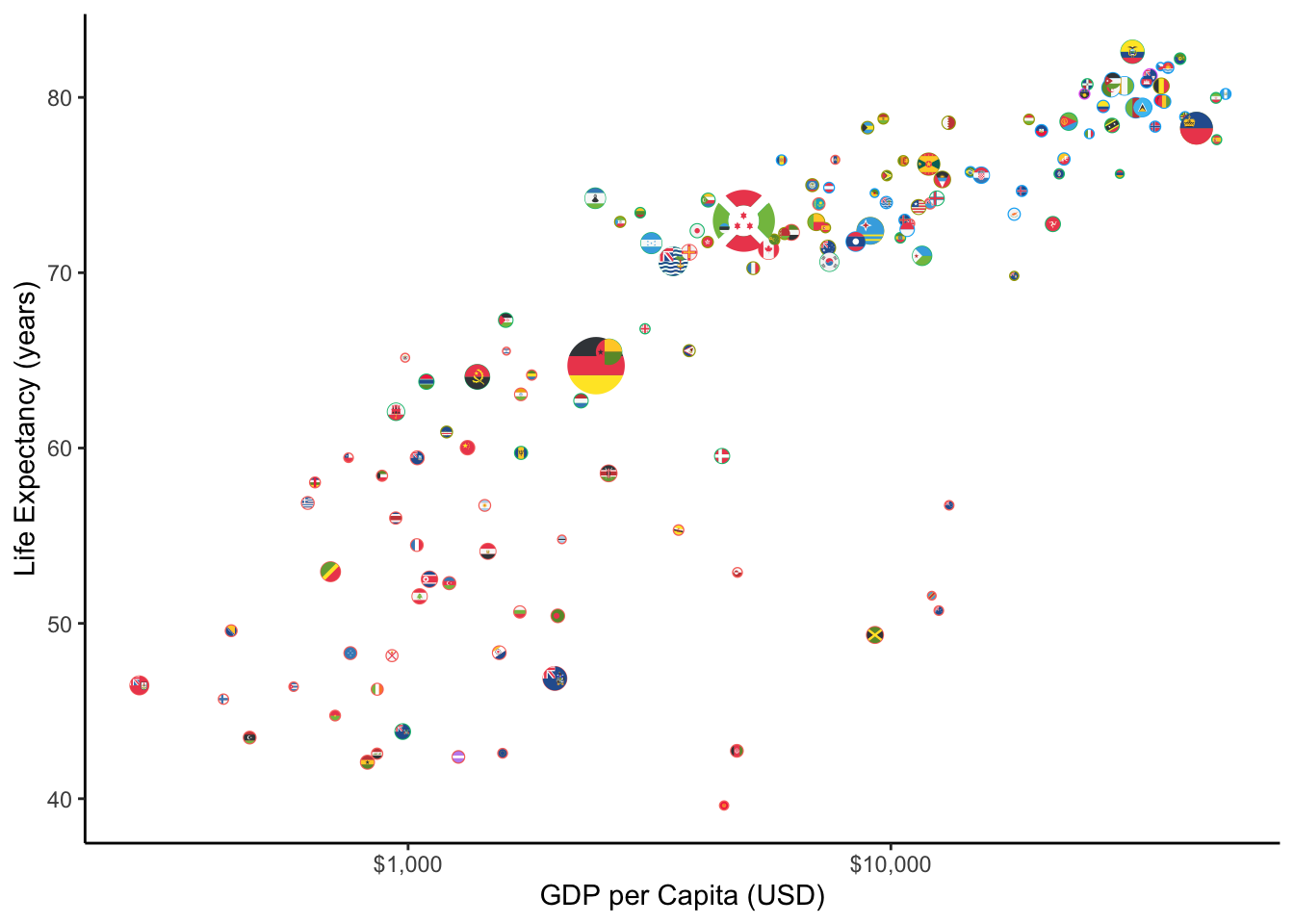### plotly

We can also make our plot a bit more interactive (on web only of course!) using the `ggplotly` package, which allows `ggplot2` to interface with a javascript library called `plotly`.I am plotting our graph from above, not the flags, since `ggflags` has not been configured for `plotly` yet.

``````# install.packages("plotly") # do this only once
library(plotly)``````
``ggplotly(p3)``

### Better barplots

Another major type of plot that we may use often is a barplot. Suppose we want to show the GDP per Capita of the top 20 countries in 2007. If I were to plot `country` on the `x` axis and `gdpPercap` on the `y` axis with `geom_col`,Using `geom_col()` allows you to specify an `x` and a `y` variable. `geom_bar()` only plots the `counts` of each value on the `x` axis. If we had done `+aes(x = country)+geom_bar()`, it would plot the number of observations of each `country` in the data, not what we want!

I get the following mess:

``````ggplot(gap2007)+
aes(x = country,
y = gdpPercap,
fill = continent)+
geom_col()``````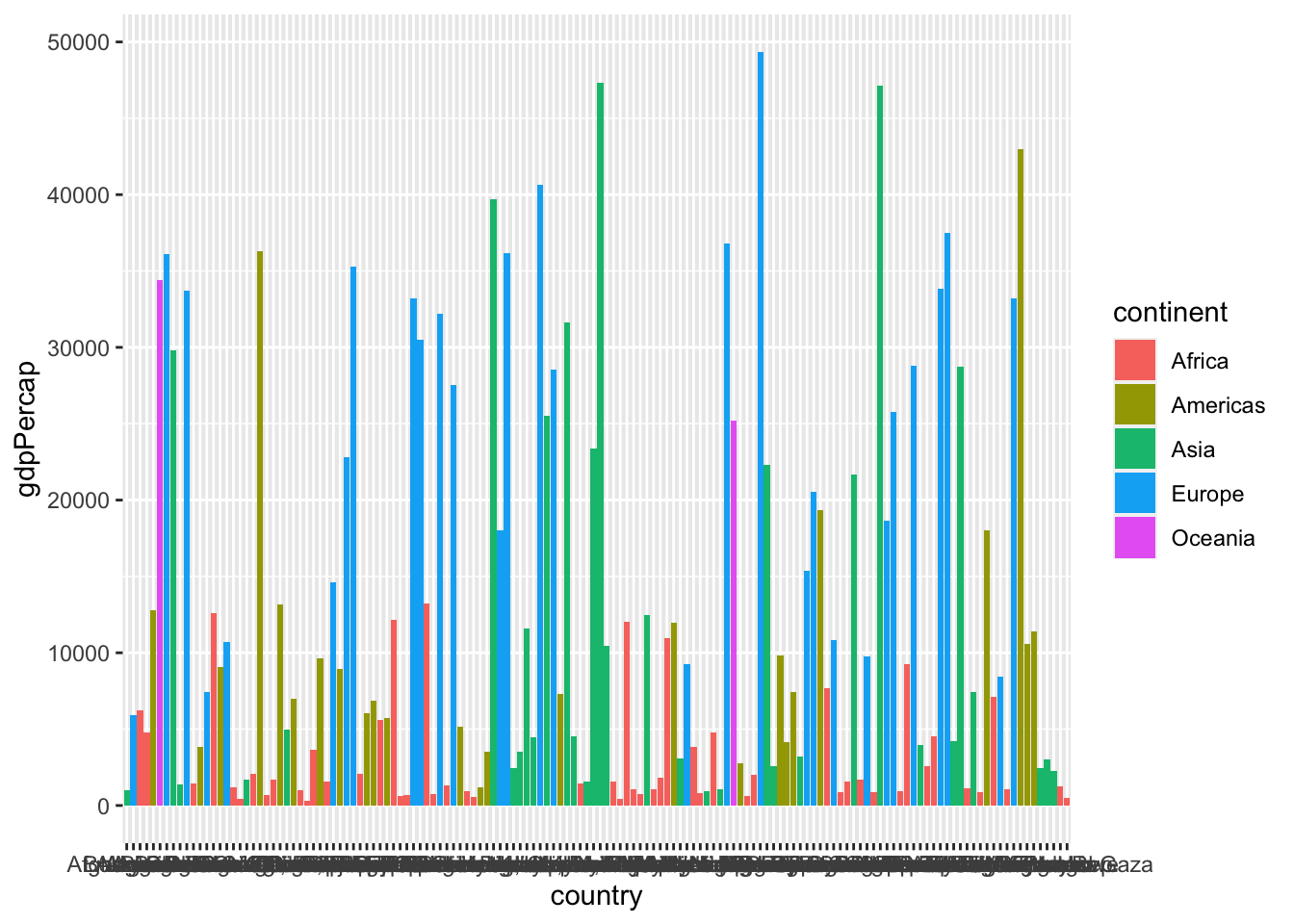So let’s `filter`You learn about this in class 1.4

arrange our data in `desc`ending order by `gdpPercap`:

``````gap2007 %>%
arrange(desc(gdpPercap))``````
``````## # A tibble: 142 x 6
##    country          continent  year lifeExp       pop gdpPercap
##    <fct>            <fct>     <int>   <dbl>     <int>     <dbl>
##  1 Norway           Europe     2007    80.2   4627926    49357.
##  2 Kuwait           Asia       2007    77.6   2505559    47307.
##  3 Singapore        Asia       2007    80.0   4553009    47143.
##  4 United States    Americas   2007    78.2 301139947    42952.
##  5 Ireland          Europe     2007    78.9   4109086    40676.
##  6 Hong Kong, China Asia       2007    82.2   6980412    39725.
##  7 Switzerland      Europe     2007    81.7   7554661    37506.
##  8 Netherlands      Europe     2007    79.8  16570613    36798.
##  9 Canada           Americas   2007    80.7  33390141    36319.
## 10 Iceland          Europe     2007    81.8    301931    36181.
## # … with 132 more rows``````

We only want the top 20 observations, so lets `slice` to extract just rows `1:20`. Then we’ll pipe it into our plot:

``````bar<-gap2007 %>%
arrange(desc(gdpPercap)) %>%
slice(1:20) %>%
ggplot(data = .)+
aes(x = country,
y = gdpPercap,
fill = continent)+
geom_col()
bar``````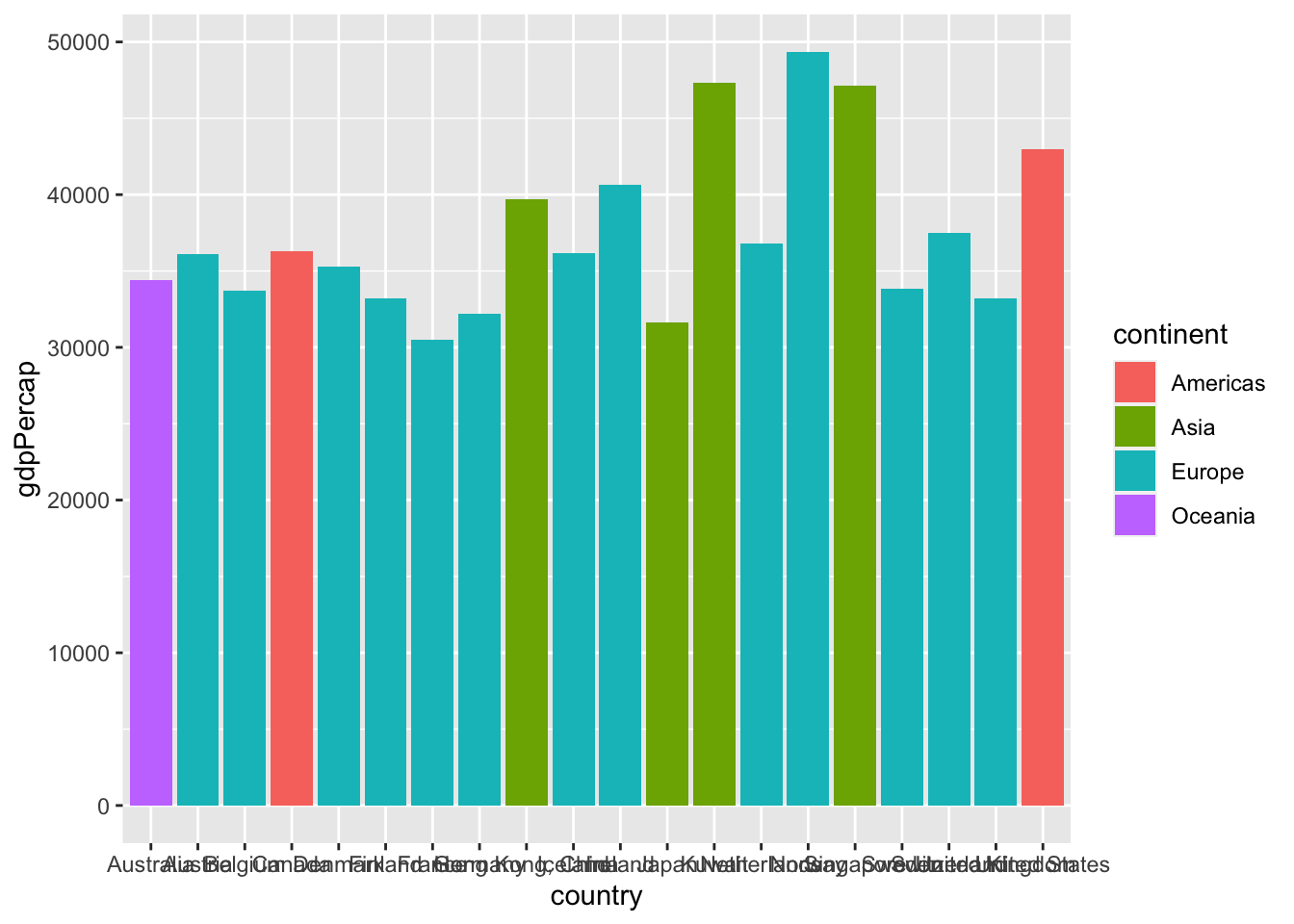Now that’s closer to what we wanted! But here are a few more tips and tricks to make it better. First, let’s flip the axes to be able to read the countries better, using `coord_flip()`

``bar+coord_flip()``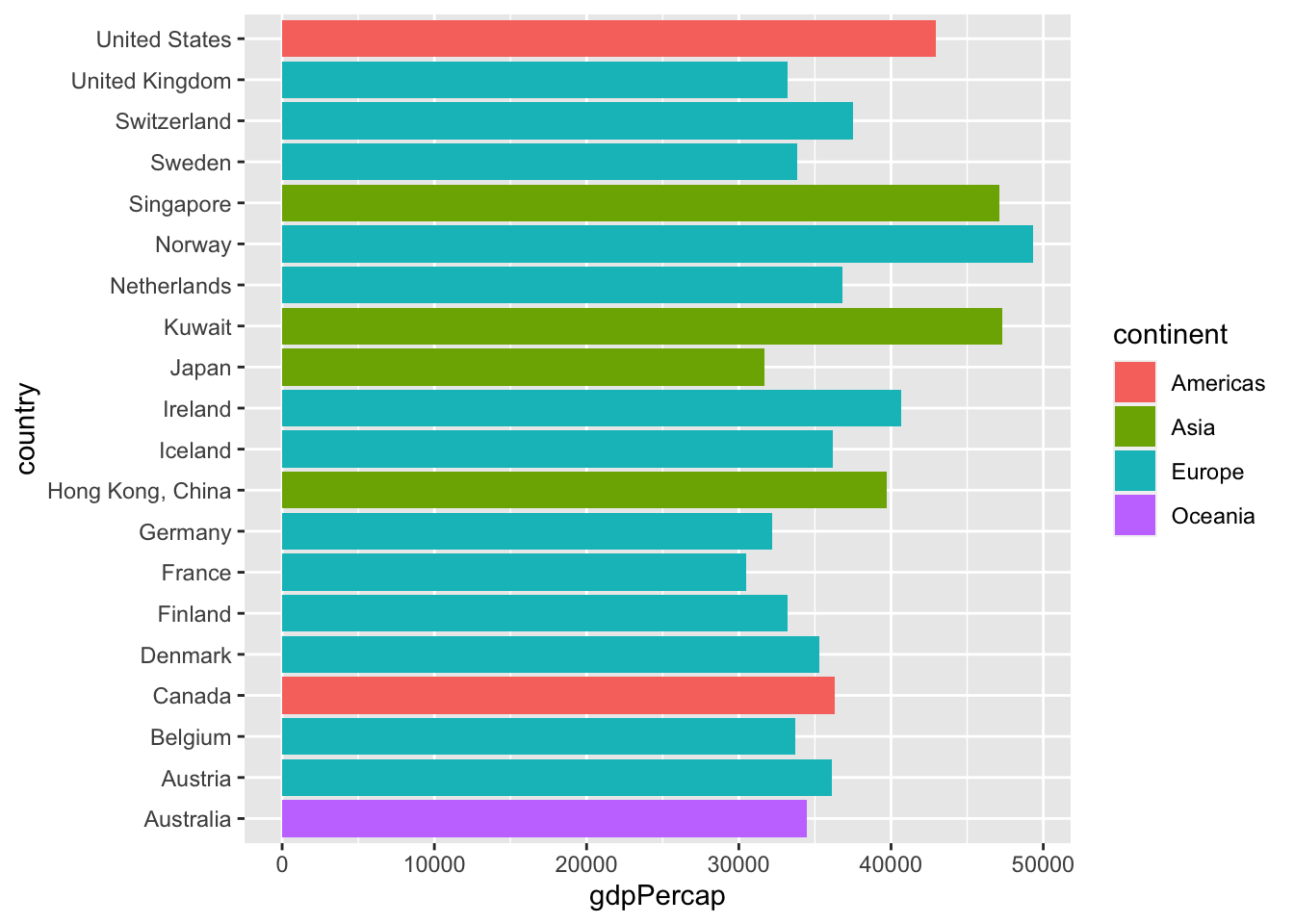One other useful thing to know would be how to display the bars in numerical order (from largest `gdpPercap` to smalleset `gdpPercap`) so we can get a clear ranking of countries. To do this, we are going to make use of another `tidyverse` package called `forcats` (dealing with `factors`), specifically the function `fct_reorder()`, which reorders a `factor` variable (our `country`) by the values of some other variable (our `gdpPercap`). We need to do this to our `x` variable `aes`thetic:

``````bar2<-gap2007 %>%
arrange(desc(gdpPercap)) %>%
slice(1:20) %>%
ggplot(data = .)+
aes(x = forcats::fct_reorder(country, gdpPercap), #<<
y = gdpPercap,
fill = continent)+
geom_col()+
coord_flip()
bar2``````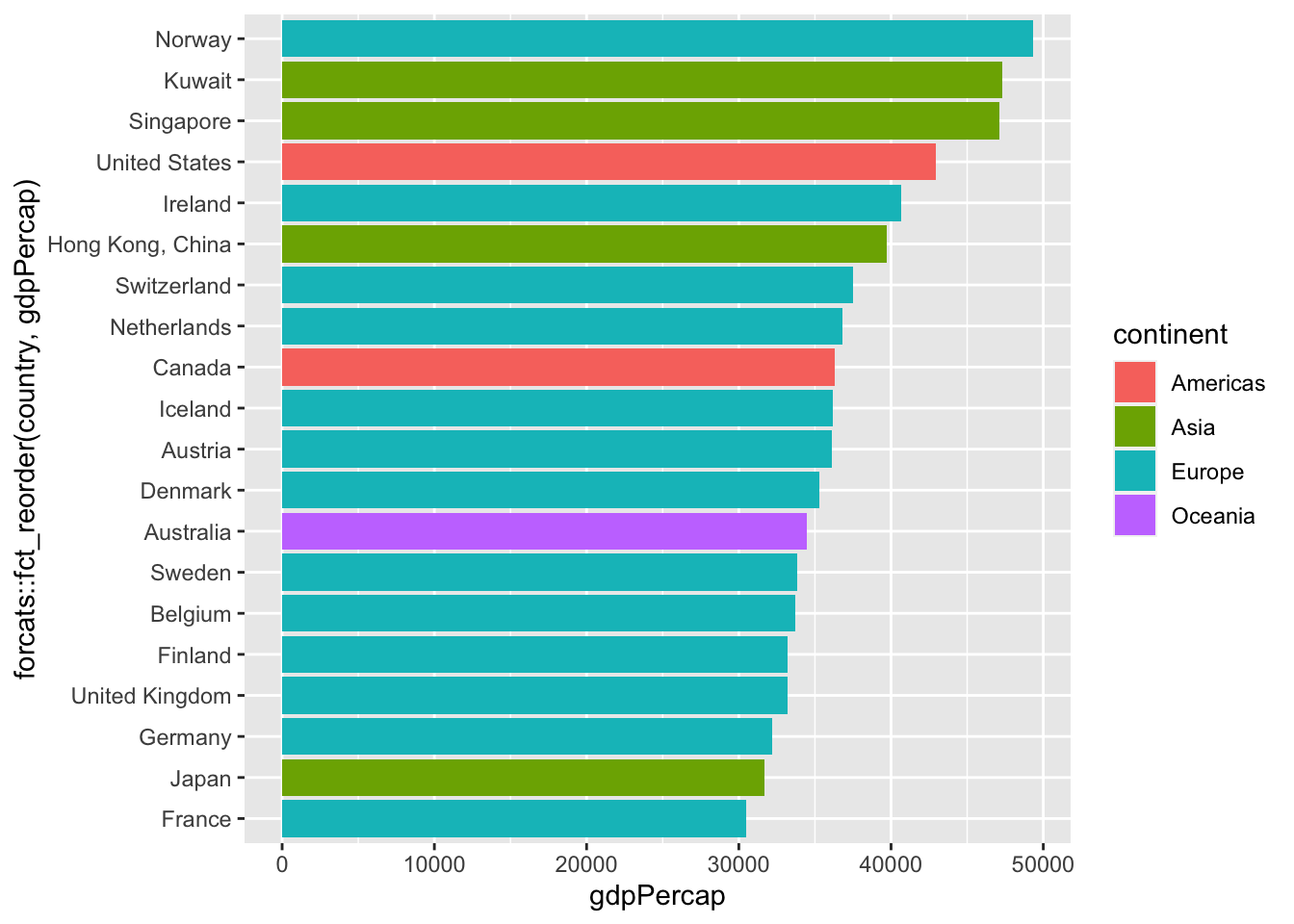This is already looking good. Here’s another creative way to depict the same thing in a more visually-striking way. Instead of using `geom_bar()`, let’s combine `geom_flag` (to serve as end points) and `geom_segment()`Note because we are defining an `x` `aes`thetic again for this, we need to make sure `x` is also the reordered list of countries, so note I am doing the whole `fct_reorder()` again.

(line segments) to make the following version:

``````bar3<-gap2007 %>%
arrange(desc(gdpPercap)) %>%
slice(1:20) %>%
ggplot(data = .)+
aes(x = forcats::fct_reorder(country, gdpPercap), #<<
y = gdpPercap,
fill = continent)+
geom_segment(aes(x = forcats::fct_reorder(country, gdpPercap), #<<
y = 0, #<<
xend = country, #<<
yend = gdpPercap, #<<
color = continent), #<<
size = 1)+ #<<
geom_flag(aes(country = country))+ #<<
coord_flip()
bar3``````